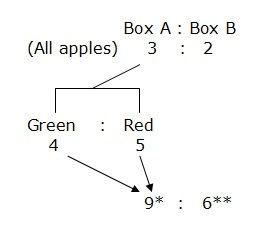This blog is managed by Song Hock Chye, author of Improve Your Thinking Skills in Maths (P1-P3 series), which is published and distributed by EPH.

## Wednesday, September 12, 2012

### Red Swastika Sch 2011 PSLE Math Prelim Paper 2 Q10

Katelyn had some green, red and blue ribbons. She had 25% more green ribbons than red ribbons and 20% less blue ribbons than red ones.

a) What is the ratio of red ribbons to green ribbons to blue ribbons?

b) Katelyn exchanges all her blue ribbons for some red and green ribbons. She now has an equal number of red and green ribbons. How many more green than red ribbons did she have at first if she has 244 red ribbons now?

Solution

(a)
Red ----- 100%
Green ----- 25% more than 100% = 125%
Blue ----- 20% less than 100% = 80%

Red : Green : Blue
100 : 125 : 80
20 : 25 : 16

Answer: 20 : 25 : 16

(b)
Before Katelyn exchanges,
Red : Green : Blue
20 : 25 : 16

Blue exchanged for green and red will mean that 16 units of blue will be given up. Since Katelyn finally has an equal of number red and green, 5 units of the 16 units of blue will be exchanged for red first, with the remaining 11 units divided equally between red and green.

Red will receive ----- 5 units + (11 units divided by 2) = 10.5 units
Green will receive ----- 11 units divided by 2 = 5.5 units

Red ribbons now ----- 20 units + 10.5 units = 30.5 units
30.5 units ----- 244
1 unit ---- 244 divided by 30.5 = 8

In the beginning,
Green - Red
25 units - 20 units = 5 units
5 units ----- 5 x 8 = 40

Printer Friendly Version

## Tuesday, September 11, 2012

### Red Swastika Sch 2011 PSLE Math Prelim Paper 2 Q8

The ratio of the number of apples in Box A to the number of apples in Box B is 3 : 2. All the apples in Box B are green. The ratio of the number of green to the number of red apples in Box A is 4 : 5. There are 20 more green apples in Box B than in Box A. How many red apples are there?

Solution* 4 units of green apples + 5 units of red ---- 9 units in Box A
** 3 units in Box A (x 3) ---- 9 units, hence 2 units in Box B (x 3) will give 6 units.

Green Apples
Box B - Box A ----- 20
6 units - 4 units ----- 20
2 units ----- 20
1 unit ----- 20 divided by 2 = 10

Red Apples
5 units ----- 5 x 10 = 50